×#### Thank you for registering.

One of our academic counsellors will contact you within 1 working day.

Click to Chat

1800-1023-196

+91-120-4616500

CART 0

• 0

MY CART (5)

Use Coupon: CART20 and get 20% off on all online Study Material

ITEM
DETAILS
MRP
DISCOUNT
FINAL PRICE
Total Price: Rs.

There are no items in this cart.
Continue Shopping```Objective Problems of Definite Problems

Objective ProblemsQ1.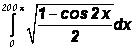(A)  0

(B) 100

(C)  200

(D) 400

Solution= –200 (cos x)π0 = –200 (–1–1) = 400

Q2. ∫2–2 min (x–[x], – x – [–x])dx,   where [.] is the greatest integer function =

(A)  0

(B) 1

(C) 2

(D) None of these.

Solution:

Let I = ∫2–2 min (x – [x], – x – x [–x])dx

= 2 ∫2–2 min (x – [x], – x – [–x])dx (function is even)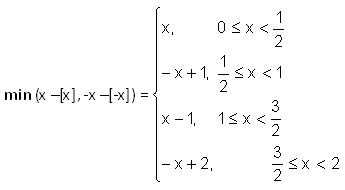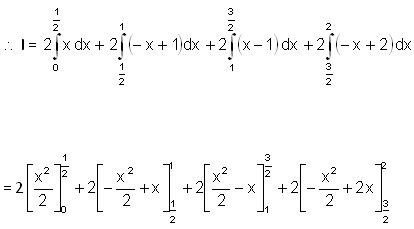= 1/4 + 1/4 + 1/4 + 1/4 = 1

Hence I = 1

Q3. (where [.] denotes greatest integer function) =

(A)  2-√2

(B)  2+√2

(C)  2√2

(D) √2

Solution: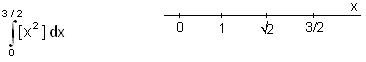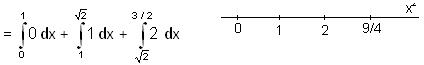= (√2 – 1) + 2 (3/2 – √2) = √2 – 1 + 3 – 2 √2 = 2 + √2 – 2 √2 = 2 – √2

Q4. Qp/20 sin 2 x in tan x dx =

(A)  2

(B) 0

(C) 1

(D) None of these.

Solution:

I = ∫π/20 sin 2x ln (tan x) dx      … (1)

I = ∫π/20 sin 2 (π/2 – x) ln tan (π/2 – x)dx

I = ∫π/20 sin 2x ln (cot x) dx     … (2)

2I = ∫π/20 sin 2x [ln (tan x) + ln (cot x)] dx = ∫π/20 sin 2x ln 1 dx = 0.

Q5. O21/2 1/x sin e/e x – 1/x o/o dx =

(A)  0

(B)  1

(C)  2

(D)  √2

Solution:

Let I =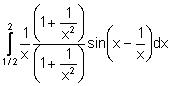Put z = x - 1/x

Then I =is odd function

Q6. The value of ∫π/20 √sin x / √sin x + √cos x is

(A) p/2

(B) p/3

(C) π/4

(D) p

Solution:

Let I = ∫π/20 √sin x / √sin x + √cos x dx

Here the lower limit is zero hence , we can replace   x by (a – x) i.e. by p/2 –x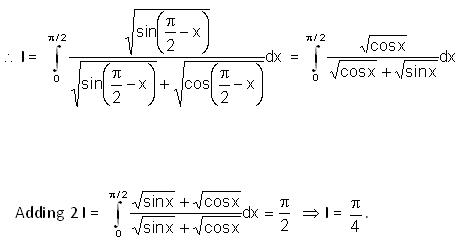Q7.=

(A) 3/p log 2

(B) 2/p log 2

(C) 3/p log 3

(D) 3/p

Solution: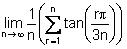r/n = x, 1/n = dx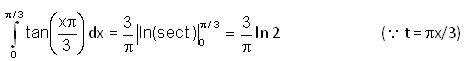Q8. The area bounded by the curve y = x (3 – x)2 , the x – axis and the ordinates of the maximum and minimum points of the curve is

(A) 2 sq. units

(B) 6 sq. units

(C) 4 sq. units

(D) 8 sq. units

Solution: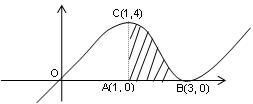y = x (3 – x)2

After solving , we get x =1 and x = 3 are points of maximum and minimum respectively.

Now the shaded region is the required region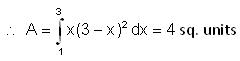Q9. What is the area of a plane figure bounded by the points of the lines max (x, y) = 1 and x2 + y2 = 1 ?

(A) p/2 sq. units

(B) p/3 sq. units

(C) p/4  sq. units

(D) p sq. units

Solution:

By definition the lines max, (x, y) = 1 means.

x = 1 and y < 1 or y = 1 and x < 1

Required areaQ10. The area bounded by the curve y = (x –1) (x – 2) (x – 3) lying between the ordinates x = 0 and x = 3 is

(A) 7/4 sq. units

(B) 4 sq. units

(C) 11/ 4 sq. units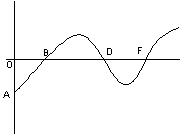(D) 3 sq. units= 9 / 4 + 1/ 4 + 1/ 4 = 11/4  sq units.
```### Course Features

• 731 Video Lectures
• Revision Notes
• Previous Year Papers
• Mind Map
• Study Planner
• NCERT Solutions
• Discussion Forum
• Test paper with Video Solution